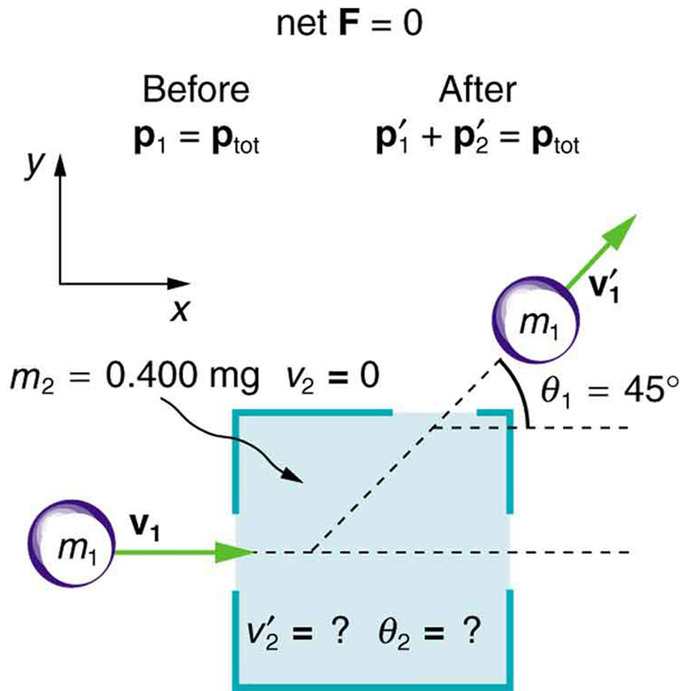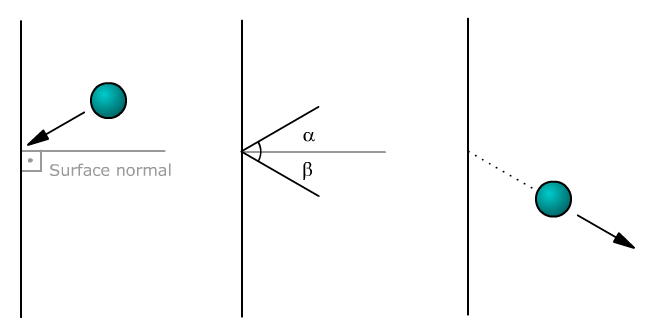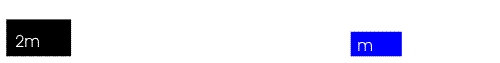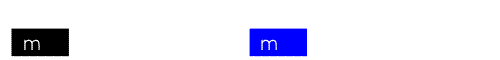## Conservation of Energy and Momentum

In an inelastic collision the total kinetic energy after the collision is not equal to the total kinetic energy before the collision.

### Learning Objectives

Assess the conservation of total momentum in an inelastic collision

### Key Takeaways

#### Key Points

• In an inelastic collision the total kinetic energy after the collision is not equal to the total kinetic energy before the collision.
• If there are no net forces at work (collision takes place on a frictionless surface and there is negligible air resistance ), there must be conservation of total momentum for the two masses.
• The variable θ is the angle between the velocity vector of the mass of interest and the x-axis in traditional Cartesian coordinate systems.

#### Key Terms

• kinetic energy: The energy possessed by an object because of its motion, equal to one half the mass of the body times the square of its velocity.
• momentum: (of a body in motion) the product of its mass and velocity.

At this point we will expand our discussion of inelastic collisions in one dimension to inelastic collisions in multiple dimensions. It is still true that the total kinetic energy after the collision is not equal to the total kinetic energy before the collision. While inelastic collisions may not conserve total kinetic energy, they do conserve total momentum.

We will consider an example problem in which one mass $(\text{m}_1)$ slides over a frictionless surface into another initially stationary mass $(\text{m}_2)$. Air resistance will be neglected. The following things are known:

$\text{m}_1 = 0.250 \text{kg}$,

$\text{m}_2 = 0.400 \text{kg}$,

$\text{v}_1=2.00\;\text{m}/\text{s}$,

$\text{v}{}'_1=1.50\;\text{m}/\text{s}$,

$\text{v}_2=0\;\text{m}/\text{s}$,

$\theta {}'_1 =45.0^\circ$,

where $\text{v}_1$ is the initial velocity of the first mass, $\text{v}{}'_1$ is the final velocity of the first mass, $\text{v}_2$ is the initial velocity of the second mass, and $\theta {}'_1$ is the angle between the velocity vector of the first mass and the x-axis.

The object is to calculate the magnitude and direction of the velocity of the second mass. After this, we will calculate whether this collision was inelastic or not.

Since there are no net forces at work (frictionless surface and negligible air resistance), there must be conservation of total momentum for the two masses. Momentum is equal to the product of mass and velocity. The initially stationary mass contributes no initial momentum. The components of velocities along the x-axis have the form $\text{v} \cdot cos \theta$, where θ is the angle between the velocity vector of the mass of interest and the x-axis.

Expressing these things mathematically:

$\text{m}_1\text{v}_1=\text{m}_1\text{v}{}'_1\cdot cos(\theta_1)+\text{m}_2\text{v}{‘}_2\cdot cos(\theta_2)$. (Eq. 2)

The components of velocities along the y-axis have the form v \cdot sin θ, where θ is the angle between the velocity vector of the mass of interest and the x-axis. By applying conservation of momentum in the y-direction we find:

$0=\text{m}_1\text{v}{}'_1\cdot sin(\theta_1)+\text{m}_2\text{v}{‘}_2\cdot sin(\theta_2)$. (Eq. 3)

If we divide Eq. 3 by Eq. 2, we will find:

$tan{\theta_2}=\frac{\text{v}{}'_1\cdot sin\theta_1}{\text{v}{}'_1cos\theta \theta _1-\text{v}_1}$(Eq. 4)

Eq. 4 can then be solved to find $\theta_2$approx. 312º.

Now let’ use Eq. 3 to solve for $\text{v}{}'_2$. Re-arranging Eq. 3, we find:

$\text{v}{}'_2=\frac{-\text{m}_1\text{v}{}'_1\cdot sin\theta_1 }{\text{m}_2\cdot sin\theta_2}$.

After plugging in our known values, we find that $\text{v}{}'_2= 0.886\;\text{m}/\text{s}$.

We can now calculate the initial and final kinetic energy of the system to see if it the same.

Initial Kinetic Energy =$\frac{1}{2}\text{m}_1\cdot \text{v}_{1}^2+\frac{1}{2}\text{m}_2\cdot \text{v}_{2}^2 = 0.5 \text{J}$.

Final Kinetic Energy = $\frac{1}{2}\text{m}_1\cdot {\text{v}{}'_{1}}^2+\frac{1}{2}\text{m}_2\cdot {\text{v}{}'_{2}}^2\approx 0.43 \text{J}$.

Since these values are not the same we know that it was an inelastic collision.Collision Example: This illustrates the example problem in which one mass collides into another mass that is initially stationary.

## Glancing Collisions

Glancing collision is a collision that takes place under a small angle, with the incident body being nearly parallel to the surface.

### Learning Objectives

Identify necessary conditions for a “glancing collision”

### Key Takeaways

#### Key Points

• Collision is short duration interaction between two bodies or more than two bodies simultaneously causing change in motion of bodies involved due to internal forces acted between them during this.
• Collisions can either be elastic, meaning they conserve both momentum and kinetic energy, or inelastic, meaning they conserve momentum but not kinetic energy.
• When dealing with an incident body that is nearly parallel to a surface, it is sometimes more useful to refer to the angle between the body and the surface, rather than that between the body and the surface normal.

#### Key Terms

• kinetic energy: The energy possessed by an object because of its motion, equal to one half the mass of the body times the square of its velocity.
• momentum: (of a body in motion) the product of its mass and velocity.
• force: A physical quantity that denotes ability to push, pull, twist or accelerate a body which is measured in a unit dimensioned in mass × distance/time² (ML/T²): SI: newton (N); CGS: dyne (dyn)

A collision is short duration interaction between two bodies or more than two bodies simultaneously causing change in motion of bodies involved due to internal forces acted between them during this. Collisions involve forces (there is a change in velocity ). The magnitude of the velocity difference at impact is called the closing speed. All collisions conserve momentum. What distinguishes different types of collisions is whether they also conserve kinetic energy. Line of impact – It is the line which is common normal for surfaces are closest or in contact during impact. This is the line along which internal force of collision acts during impact and Newton’s coefficient of restitution is defined only along this line.

When dealing with an incident body that is nearly parallel to a surface, it is sometimes more useful to refer to the angle between the body and the surface, rather than that between the body and the surface normal (see ), in other words 90° minus the angle of incidence. This small angle is called a glancing angle. Collision at glancing angle is called “glancing collision”.Collision: Object is deflected after the collision withthe surface. The angles between the body and the surface normal areindicated as α and β. The angles between the body and the surface are 90 – α and 90 – β.

Collisions can either be elastic, meaning they conserve both momentum and kinetic energy, or inelastic, meaning they conserve momentum but not kinetic energy. An inelastic collision is sometimes also called a plastic collision.

A “perfectly-inelastic” collision (also called a “perfectly-plastic” collision) is a limiting case of inelastic collision in which the two bodies stick together after impact.

The degree to which a collision is elastic or inelastic is quantified by the coefficient of restitution, a value that generally ranges between zero and one. A perfectly elastic collision has a coefficient of restitution of one; a perfectly-inelastic collision has a coefficient of restitution of zero.

## Elastic Collisions in One Dimension

An elastic collision is a collision between two or more bodies in which kinetic energy is conserved.

### Learning Objectives

Assess the relationship among the collision equations to derive elasticity

### Key Takeaways

#### Key Points

• An elastic collision will not occur if kinetic energy is converted into other forms of energy.
• While molecules do not undergo elastic collisions, atoms often undergo elastic collisions when they collide.
• If two particles are involved in an elastic collision, the velocity of the first particle after collision can be expressed as: $\text{v}_{1\text{f}} =\frac{(\text{m}_1-\text{m}_2)}{(\text{m}_2+\text{m}_1)}\text{v}_{1\text{i}}+\frac{2\cdot \text{m}_2}{(\text{m}_2+\text{m}_1)}\text{v}_{2\text{i}}$.
• If two particles are involved in an elastic collision, the velocity of the second particle after collision can be expressed as: $\text{v}_{2\text{f}} =\frac{2\cdot \text{m}_1}{(\text{m}_2+\text{m}_1)}\text{v}_{1\text{i}} +\frac{(\text{m}_2-\text{m}_1)}{(\text{m}_2+\text{m}_1)}\text{v}_{2\text{i}}$.

#### Key Terms

• elastic collision: An encounter between two bodies in which the total kinetic energy of the two bodies after the encounter is equal to their total kinetic energy before the encounter. Elastic collisions occur only if there is no net conversion of kinetic energy into other forms.
• momentum: (of a body in motion) the product of its mass and velocity.
• kinetic energy: The energy possessed by an object because of its motion, equal to one half the mass of the body times the square of its velocity.

An elastic collision is a collision between two or more bodies in which the total kinetic energy of the bodies before the collision is equal to the total kinetic energy of the bodies after the collision. An elastic collision will not occur if kinetic energy is converted into other forms of energy. It important to understand how elastic collisions work, because atoms often undergo essentially elastic collisions when they collide. On the other hand, molecules do not undergo elastic collisions when they collide. In this atom we will review case of collision between two bodies.

The mathematics of an elastic collision is best demonstrated through an example. Consider a first particle with mass $\text{m}_{1}$ and velocity $\text{v}_{1\text{i}}$ and a second particle with mass $\text{m}_{2}$ and velocity $\text{v}_{2\text{i}}$. If these two particles collide, there must be conservation of momentum before and after the collision. If we know that this is an elastic collision, there must be conservation of kinetic energy by definition. Therefore, the velocities of particles 1 and 2 after the collision ($\text{v}_{1\text{f}}$ and $\text{v}_{2\text{f}}$ respectively) will be related to the initial velocities by:

$\frac{1}{2}\text{m}_1\cdot \text{v}_{1\text{i}}^2+\frac{1}{2}\text{m}_2\cdot \text{v}_{2\text{i}}^2=\frac{1}{2}\text{m}_1\cdot \text{v}_{1\text{f}}^2+\frac{1}{2}\text{m}_2\cdot \text{v}_{2\text{f}}^2$ (due to conservation of kinetic energy)

and

$\text{m}_1\cdot \text{v}_{1\text{i}}+\text{m}_2\cdot \text{v}_{2\text{i}}=\text{m}_1\cdot \text{v}_{1\text{f}}+\text{m}_2\cdot \text{v}_{2\text{f}}$ (due to conservation of momentum).

Since we have two equations, we are able to solve for any two unknown variables. In our case, we will solve for the final velocities of the two particles.

By grouping like terms and canceling out the ½ terms, we can rewrite our conservation of kinetic energy equation as:

$\text{m}_1\cdot (\text{v}_{1\text{i}}^2-\text{v}_{1\text{f}}^2) = \text{m}_2\cdot (\text{v}_{2\text{f}}^2-\text{v}_{2\text{i}}^2)$. (Eq.1)

By grouping like terms from our conservation of momentum equation we can find:

$\text{m}_1\cdot (\text{v}_{1\text{i}}-\text{v}_{1\text{f}}) = \text{m}_2\cdot (\text{v}_{2\text{f}}-\text{v}_{2\text{i}})$. (Eq. 2)

If we then divide Eq. 1 by Eq. 2 and perform some cancelations we will find:

$\text{v}_{1\text{i}} + \text{v}_{1\text{f}} = \text{v}_{2\text{f}} + \text{v}_{2\text{i}}$. (Eq. 3)

We can solve for $\text{v}_{1\text{f}}$ as:

$\text{v}_{1\text{f}} = \text{v}_{2\text{f}} + \text{v}_{2\text{i}}-\text{v}_{1\text{i}}$. (Eq. 4)

At this point we see that $\text{v}_{2\text{f}}$ is still an unknown variable. So we can fix this by plugging Eq. 4 into our initial conservation of momentum equation. Our conservation of momentum equation with Eq. 4 substituted in looks like:

$\text{m}_1\cdot \text{v}_{1\text{i}}+\text{m}_2\cdot \text{v}_{2\text{i}}=\text{m}_1\cdot(\text{v}_{2\text{f}} + \text{v}_{2\text{i}}-\text{v}_{1\text{i}})+\text{m}_2\cdot \text{v}_{2\text{f}}$. (Eq.5)

After doing a little bit of algebra on Eq. 5 we find:

$\text{v}_{2\text{f}} =\frac{2\cdot \text{m}_1}{(\text{m}_2+\text{m}_1)}\text{v}_{1\text{i}} +\frac{(\text{m}_2-\text{m}_1)}{(\text{m}_2+\text{m}_1)}\text{v}_{2\text{i}}$. (Eq.6)

At this point we have successfully solved for the final velocity of the second particle. We still need to solve for the velocity of the first particle, so let us do that by plugging Eq. 6 into Eq. 4.

$\text{v}_{1\text{f}} = [\frac{2\cdot \text{m}_1}{(\text{m}_2+\text{m}_1)}\text{v}_{1\text{i}} +\frac{(\text{m}_2-\text{m}_1)}{(\text{m}_2+\text{m}_1)}\text{v}_{2\text{i}}] + \text{v}_{2\text{i}}-\text{v}_{1\text{i}}$. (Eq. 7)

After performing some algebraic manipulation of Eq. 7, we finally find:

$\text{v}_{1\text{f}} =\frac{(\text{m}_1-\text{m}_2)}{(\text{m}_2+\text{m}_1)}\text{v}_{1\text{i}}+\frac{2\cdot \text{m}_2}{(\text{m}_2+\text{m}_1)}\text{v}_{2\text{i}}$. (Eq. 8)Elastic Collision of Two Unequal Masses: In this animation, two unequal masses collide and recoil.

## Elastic Collisions in Multiple Dimensions

To solve a two dimensional elastic collision problem, decompose the velocity components of the masses along perpendicular axes.

### Learning Objectives

Construct an equation for elastic collision

### Key Takeaways

#### Key Points

• If an elastic collision occurs in two dimensions, the colliding masses can travel side to side after the collision.
• By defining the x- axis to be along the direction of the incoming particle, we can simplify the defining equations.
• The general approach to finding the defining equations for an n-dimensional elastic collision problem is to apply conservation of momentum in each of the n- dimensions. You can generate an additional equation by utilizing conservation of kinetic energy.

#### Key Terms

• kinetic energy: The energy possessed by an object because of its motion, equal to one half the mass of the body times the square of its velocity.
• dimension: A measure of spatial extent in a particular direction, such as height, width or breadth, or depth.
• momentum: (of a body in motion) the product of its mass and velocity.

### Overview

As stated previously, there is conservation of total kinetic energy before and after an elastic collision. If an elastic collision occurs in two dimensions, the colliding masses can travel side to side after the collision (not just along the same line as in a one dimensional collision). The general approach to solving a two dimensional elastic collision problem is to choose a coordinate system in which the velocity components of the masses can be decomposed along perpendicular axes.

Collisions in Multiple Dimensions: A brief introduction to problem solving of collisions in two dimensions using the law of conservation of momentum.

### Example

In this example, we consider only point masses. These are structure-less particles that cannot spin or rotate. We will consider a case in which no outside forces are acting on the system, meaning that momentum is conserved. We will consider a situation in which one particle is initially at rest. This situation is illustrated in.Illustration of Elastic Collision in Two Dimensions: In this illustration, we see the initial and final configurations of two masses that undergo an elastic collision in two dimensions.

By defining the x-axis to be along the direction of the incoming particle, we save ourselves time in breaking that velocity vector into its x- and y- components. Now let us consider conservation of momentum in the x direction:

$\text{p}_{1\text{x}}+\text{p}_{2\text{x}}=\text{p}{}'_{1\text{x}}+\text{p}{}'_{2\text{x}}$ (Eq. 1)

In Eq. 1, the initial momentum of the incoming particle is represented by p1x, the initial momentum of the stationary particle is represented by p2x, the final momentum of the incoming particle is represented by p’1x. and the final momentum of the initially stationary particle is represented by p’2x.

We can expand Eq. 1 by taking into account that momentum is equal to the product of mass and velocity. Also, we know that p2x = 0 because the initial velocity of the stationary particle is 0.

The components of velocities along the x-axis have the form $\text{v} \cdot cos \theta$, where θ is the angle between the velocity vector of the particle of interest and the x-axis.

Therefore:

$\text{m}_1\text{v}_1=\text{m}_1\text{v}{}'_1\cdot cos(\theta_1)+\text{m}_2\text{v}{‘}_2\cdot cos(\theta_2)$ (Eq. 2)

The components of velocities along the y-axis have the form $\text{v} \cdot sin \theta$, where θ is the angle between the velocity vector of the particle of interest (denoted in the following equations by subscript 1 or 2) and the x-axis. We can apply conservation of momentum in the y-direction in a similar way to yield:

$0=\text{m}_1\text{v}{}'_1\cdot sin(\theta_1)+\text{m}_2\text{v}{‘}_2\cdot sin(\theta_2)$ (Eq. 3)

In finding Eq. 3, it was taken into consideration that the incoming particle had no component of velocity along the y-axis.

### Solving for Two Unknowns

Now we have gotten to a point where we have two equations, this means that we can solve for any two unknowns that we want. We also know that because the collision is elastic that there must be conservation of kinetic energy before and after the collision. This means that we may also write Eq. 4, which gives us three equations to solve for three unknowns:

$\frac{1}{2}\text{m}_1\cdot {\text{v}_{1}}^2+\frac{1}{2}\text{m}_2\cdot {\text{v}_{2}}^2=\frac{1}{2}\text{m}_1\cdot {\text{v}{}'_{1}}^2+\frac{1}{2}\text{m}_2\cdot {\text{v}{}'_{2}}^2$

The general approach to finding the defining equations for an n-dimensional elastic collision problem is to apply conservation of momentum in each of the n- dimensions. You can generate an additional equation by utilizing conservation of kinetic energy.

## Inelastic Collisions in One Dimension

Collisions may be classified as either inelastic or elastic collisions based on how energy is conserved in the collision.

### Learning Objectives

Distinguish examples of inelastic collision from elastic collisions

### Key Takeaways

#### Key Points

• In an inelastic collision, the total kinetic energy after the collision is not equal to the total kinetic energy before the collision.
• While inelastic collisions may not conserve total kinetic energy, they do conserve total momentum.
• A perfectly inelastic collision happens when the maximum amount of kinetic energy in a system is lost.

#### Key Terms

• kinetic energy: The energy possessed by an object because of its motion, equal to one half the mass of the body times the square of its velocity.
• degrees of freedom: A degree of freedom is an independent physical parameter, often called a dimension, in the formal description of the state of a physical system. The set of all dimensions of a system is known as a phase space.

### Overview

In an inelastic collision the total kinetic energy after the collision is not equal to the total kinetic energy before the collision. This is in contrast to an elastic collision in which conservation of total kinetic energy applies. While inelastic collisions may not conserve total kinetic energy, they do conserve total momentum.

### Collisions

If two objects collide, there are many ways that kinetic energy can be transformed into other forms of energy. For example, in the collision of macroscopic bodies, some kinetic energy is turned into vibrational energy of the constituent atoms. This causes a heating effect and results in deformation of the bodies. Another example in which kinetic energy is transformed into another form of energy is when the molecules of a gas or liquid collide. When this happens, kinetic energy is often exchanged between the molecules’ translational motion and their internal degrees of freedom.

A perfectly inelastic collision happens when the maximum amount of kinetic energy in a system is lost. In such a collision, the colliding particles stick together. The kinetic energy is used on the bonding energy of the two bodies.

### Sliding Block Example

Let us consider an example of a two-body sliding block system. The first block slides into the second (initially stationary block). In this perfectly inelastic collision, the first block bonds completely to the second block as shown. We assume that the surface over which the blocks slide has no friction. We also assume that there is no air resistance. If the surface had friction or if there was air resistance, one would have to account for the bodies’ momentum that would be transferred to the surface and/or air.Inelastic Collision: In this animation, one mass collides into another initially stationary mass in a perfectly inelastic collision.

Writing about the equation for conservation of momentum, one finds:

$\text{m}_\text{a} \text{u}_\text{a} + \text{m}_\text{b} \text{u}_\text{b} = \left( \text{m}_\text{a} + \text{m}_\text{b} \right) \text{v}$

where mais the mass of the incoming block, ua is the velocity of the incoming block, mbis the mass of the initially stationary block, ubis the velocity of initially stationary block (0 m/s), and v is the final velocity the two body system. Solving for the final velocity,

$\text{v}=\frac{\text{m}_\text{a} \text{u}_\text{a} + \text{m}_\text{b} \text{u}_\text{b}}{\text{m}_\text{a} + \text{m}_\text{b}}$.

Taking into account that the blocks have the same mass and that the one of the blocks is initially stationary, the expression for the final velocity of the system may be defined as:

$\text{v}=\frac{\text{u}_\text{a} }{2}$.

## Inelastic Collisions in Multiple Dimensions

While inelastic collisions may not conserve total kinetic energy, they do conserve total momentum.

### Learning Objectives

Relate inelastic collision multiple dimension equations to the one dimension collisions you learned earlier

### Key Takeaways

#### Key Points

• In an inelastic collision the total kinetic energy after the collision is not equal to the total kinetic energy before the collision.
• If there are no net forces at work (i.e., collision takes place on a frictionless surface and there is negligible air resistance ), there must be conservation of total momentum for the two masses.
• The variable θ is the angle between the velocity vector of the mass of interest and the x-axis in traditional Cartesian coordinate systems.

#### Key Terms

• momentum: (of a body in motion) the product of its mass and velocity.
• kinetic energy: The energy possessed by an object because of its motion, equal to one half the mass of the body times the square of its velocity.
• friction: A force that resists the relative motion or tendency to such motion of two bodies in contact.

At this point we will expand our discussion of inelastic collisions in one dimension to inelastic collisions in multiple dimensions. It is still true that the total kinetic energy after the collision is not equal to the total kinetic energy before the collision. While inelastic collisions may not conserve total kinetic energy, they do conserve total momentum.

Examples of Collisions

We will consider an example problem, illustrated in, in which one mass ($\text{m}_1$) slides over a frictionless surface into another initially stationary mass ($\text{m}_2$). Air resistance will be neglected. The following quantities are known:Collision Example: This illustrates the example problem in which one mass collides into another mass that is initially stationary.

$\text{m}_1$ = 0.250 kg,

$\text{m}_2$ = 0.400 kg,

$\text{v}_1$ = 2.00 m/s,

$\text{v}{}'_1$ = 1.50 m/s,

$\text{v}_2$= 0 m/s,

$\theta{}'_1$ = 45.0º,

where $\text{v}_1$ is the initial velocity of the first mass, $\text{}v{}'_1$ is the final velocity of the first mass, $\text{v}_2$is the initial velocity of the second mass, and $\theta{}'_1$ is the angle between the velocity vector of the first mass and the x-axis.

The object is to calculate the magnitude and direction of the velocity of the second mass. After this, we will calculate whether this collision was inelastic or not.

Since there are no net forces at work (frictionless surface and negligible air resistance), there must be conservation of total momentum for the two masses. Momentum is equal to the product of mass and velocity. The (initially) stationary mass contributes no initial momentum. The components of velocities along the x-axis have the form $\text{v} \cdot cos \theta$, where θ is the angle between the velocity vector of the mass of interest and the x-axis.

Expressing these things mathematically:

$\text{m}_1\text{v}_1=\text{m}_1\text{v}{}'_1\cdot cos(\theta_1)+\text{m}_2\text{v}{‘}_2\cdot cos(\theta_2)$ (Eq. 2).

The components of velocities along the y-axis have the form v \cdot sin θ, where θ is the angle between the velocity vector of the mass of interest and the x-axis. By applying conservation of momentum in the y-direction we find:

$0=\text{m}_1\text{v}{}'_1\cdot sin(\theta_1)+\text{m}_2\text{v}{‘}_2\cdot sin(\theta_2)$ (Eq. 3).

If we divide Eq. 3 by Eq. 2, we will find:

$tan{\theta_2}=\frac{\text{v}{}'_1\cdot sin\theta_1}{\text{v}{}'_1cos\theta \theta _1-\text{v}_1}$ (Eq. 4).

Eq. 4 can then be solved to find $\theta_2 \approx 312^{\circ}$.

Now let’ use Eq. 3 to solve for $\text{v}{}'_2$. Re-arranging Eq. 3, we find:

$\text{v}{}'_2=\frac{-\text{m}_1\text{v}{}'_1\cdot sin\theta_1 }{\text{m}_2\cdot sin\theta_2}$.

After plugging in our known values, we find that $\text{v}{}'_2$= 0.886m/s. We can now calculate the initial and final kinetic energy of the system to see if it the same.

Initial Kinetic Energy = $\frac{1}{2}\text{m}_1\cdot \text{v}_{1}^2+\frac{1}{2}\text{m}_2\cdot \text{v}_{2}^2$ = 0.5 J.

Final Kinetic Energy = $\frac{1}{2}\text{m}_1\cdot {\text{v}{}'_1}^2+\frac{1}{2}\text{m}_2\cdot {\text{v}{}'_2}^2 \approx$ 0.43 J

As these values are not the same, we know this was an inelastic collision.